# A Visual Look at JVM Stacks and Frames

Given the background information of the previous lesson, let’s take a visual look at how the JVM stack and stack frames work by going back to our recursive sum function from the previous lesson.

Before the `sum` function is initially called, the only thing on the call stack is the application’s `main` method, as shown in Figure [fig:mainOnStack].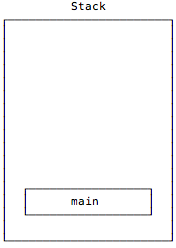Then `main` calls `sum` with `List(1,2,3)`, which I show in Figure [fig:firstSumCall] without the “List” to keep things simple.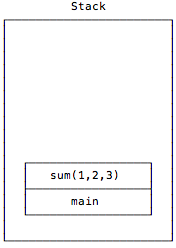The data that’s given to `sum` matches its second `case` expression, and in my pseudocode, that expression evaluates to this:

``````return 1 + sum(2,3)
``````

Next, when a new instance of `sum` is called with `List(2,3)`, the stack looks as shown in Figure [fig:theSecondSumCall].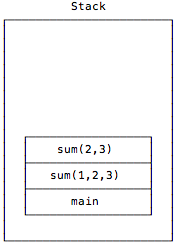Again the second `case` expression is matched inside of `sum`, and it evaluates to this:

``````return 2 + sum(3)
``````

When a new instance of `sum` is called with the input parameter `List(3)`, the stack looks like Figure [fig:theThirdSumCall].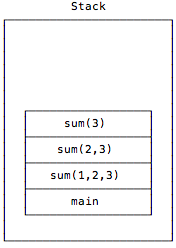Again the second `case` expression is matched, and that code evaluates to this:

``````return 3 + sum(Nil)
``````

Finally, another instance of `sum` is called with the input parameter `List()` — also known as `Nil` — and the stack now looks like Figure [fig:theFinalSumCall].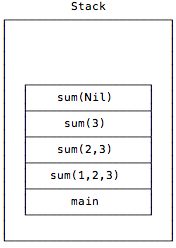This time, when `sum(Nil)` is called, the first `case` expression is matched:

``````case Nil => 0
``````

That pattern match causes this `sum` instance to return `0`, and when it does, the call stack unwinds and the stack frames are popped off of the stack, as shown in the series of images in Figure [fig:callStackUnwinding222].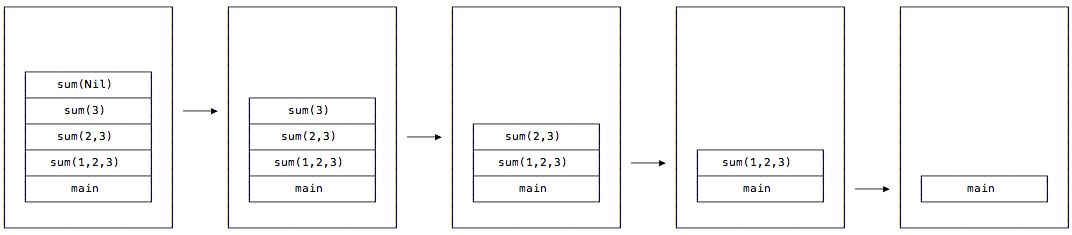In this process, as each `sum` call returns, its frame is popped off of the stack, and when the recursion completely ends, the main method is the only frame left on the call stack. (The value `6` is also returned by the first `sum` invocation to the place where it was called in the `main` method.)

I hope that gives you a good idea of how recursive function calls are pushed-on and popped-off the JVM call stack.

If you want to explore this in code, you can also see the series of `sum` stack calls by modifying the `sum` function. To do this, add a couple of lines of code to the `Nil` case to print out stack trace information when that `case` is reached:

``````def sum(list: List[Int]): Int = list match {
case Nil => {
// this manually creates a stack trace
stackTraceAsArray.foreach(println)
// return 0 as before
0
}
case x :: xs => x + sum(xs)
}
``````

Now, if you call `sum` with a list that goes from 1 to 5:

``````val list = List.range(1, 5)
sum(list)
``````

you’ll get this output when the `Nil` case is reached:

``````java.lang.Thread.getStackTrace(Thread.java:1588)
recursion.SumWithStackDump\$.sum(SumWithStackDump.scala:19)
recursion.SumWithStackDump\$.sum(SumWithStackDump.scala:19)
recursion.SumWithStackDump\$.sum(SumWithStackDump.scala:19)
recursion.SumWithStackDump\$.sum(SumWithStackDump.scala:19)
recursion.SumWithStackDump\$.sum(SumWithStackDump.scala:19)
``````

While that output isn’t too exciting, it shows that when the stack dump is manually triggered when the `Nil` case is reached, the `sum` function is on the stack five times. You can verify that this is correct by repeating the test with a `List` that has three elements, in which case you’ll see the `sum` function referenced only three times in the output:

``````java.lang.Thread.getStackTrace(Thread.java:1588)
recursion.SumWithStackDump\$.sum(SumWithStackDump.scala:13)
recursion.SumWithStackDump\$.sum(SumWithStackDump.scala:19)
recursion.SumWithStackDump\$.sum(SumWithStackDump.scala:19)
``````

Clearly the `sum` function is being added to the stack over and over again, once for each call.

I encourage you to try this on your own to become comfortable with what’s happening.

I hope this little dive into the JVM stack and stack frames helps to explain our current problem with “basic recursion.” As mentioned, if I try to pass a `List` with 10,000 elements into the current recursive `sum` function, it will generate a `StackOverflowError`. Because we’re trying to write bulletproof programs, this isn’t good.

Now that we looked at (a) basic recursion with the `sum` function, (b) how that works with stacks and stack frames in the last two lessons, and (c) how basic recursion can throw a `StackOverflowError` with large data sets, the next lesson shows how to fix these problems with something called “tail recursion.”

I didn’t get into all of the nitty-gritty details about the stack and stack frames in this lesson. If you want to learn more about the stack, here are some excellent resources:

One more thing: Viewing and setting the JVM stack size

“Well,” you say, “these days computers have crazy amounts of memory. Why is this such a problem?”

According to this Oracle document, with Java 6 the default stack size was very low: 1,024k on both Linux and Windows.

I encourage you to check the JVM stack size on your favorite computing platform(s). One way to check it is with a command like this on a Unix-based system:

``````java -XX:+PrintFlagsFinal -version | grep -i stack
``````

When I do this on my current Mac OS X system, I see that the `ThreadStackSize` is `1024`. I dug through this oracle.com documentation to find that this “1024” means “1,024 Kbytes”.

It’s important to know that you can also control the JVM stack size with the `-Xss` command line option:

``````\$ java -Xss 1M ... (the rest of your command line here)
``````

That command sets the stack size to one megabyte. You specify the memory size attribute as `m` or `M` after the numeric value to get megabytes, as in `1m` or `1M` for one megabyte.

Use `g` or `G` to specify the size in gigabytes, but if you’re trying to use many MB or GB for the stack size, you’re doing something wrong. You may need this gigabytes option for the `Xmx` option, but you should never need it for this `Xss` attribute.

The `Xss` option can be helpful if you run into a `StackOverflowError` — although the next lesson on tail recursion is intended to help you from ever needing this command line option.

More JVM memory settings

As a final note, you can find more options for controlling Java application memory use by looking at the output of the `java -X` command:

``````\$ java -X
``````

If you dig through the output of that command, you’ll find that the command-line arguments specifically related to Java application memory use are:

``````-Xms  set initial Java heap size
-Xmx  set maximum Java heap size
-Xss  set java thread stack size
``````

You can use these parameters on the java command line like this:

``````java -Xms64m -Xmx1G myapp.jar
``````

As before, valid memory values end with `m` or `M` for megabytes, and `g` or `G` for gigabytes:

``````-Xms64m or -Xms64M
-Xmx1g or -Xmx1G
``````